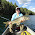Friday, 2 August 2013

The Maths behind Sled kites

When we did our Sled Kite project it involved maths: measuring, working with percentages and ratios.  However, it was a maths lesson they didn't mind if it meant they ended up with a kite.  With this in mind, I thought I would look into all the maths I could think of that relate to kite making!  So here goes...

How to work out the measurements for your kite if you want to make a different size kite:

If you look at the photo below it should help you understand what I mean when I say:

The 25cm in our template is the height of our kite, we will use as our 100% mark.

The two bits at the top and the line across the bottom ( all of which are 7.5cm in the original template) is 30% of the 25cm (100% of your kite's height)

Then you have 10% up the top left side

28% up the top right side.  Like this...You could ask the children to work out the other sides as ratios and fractions if you like.

Once you have done this you will see that you have drawn lines that you don't know the exact measurements too.

Of course you could use a ruler but, as they make right angle triangles, you could also have a go at pythagoras' theorem

All you have to remember is  a+ b2 = c2

C is known as the hypotheses

So for the triangle below we have 22 + 62 = c2

Which is 22 + 62 = 6.3242

Because 22  = 4  and 62  = 36  √40 = 6.324

You can measure it with a ruler afterwards to see if you're right.

Then there is the Geometry of the kite.

What sort of shape is the template? An irregular pentagon.

What shape is the sail of the kite?  An irregular heptagon.

Draw it out as vectors.  A vector is a set of instructions for moving from one point to another in three dimensions. A line which has both magnitude and direction can represent this vector.

Trigonometry is another thing that you can try when flying any kite - kite flying makes trigonometry super fun!  It is something I am going to introduce my girls to when we do our next kite flying activity!If you want to make your own Sled kite Click  here.

1.2.3.